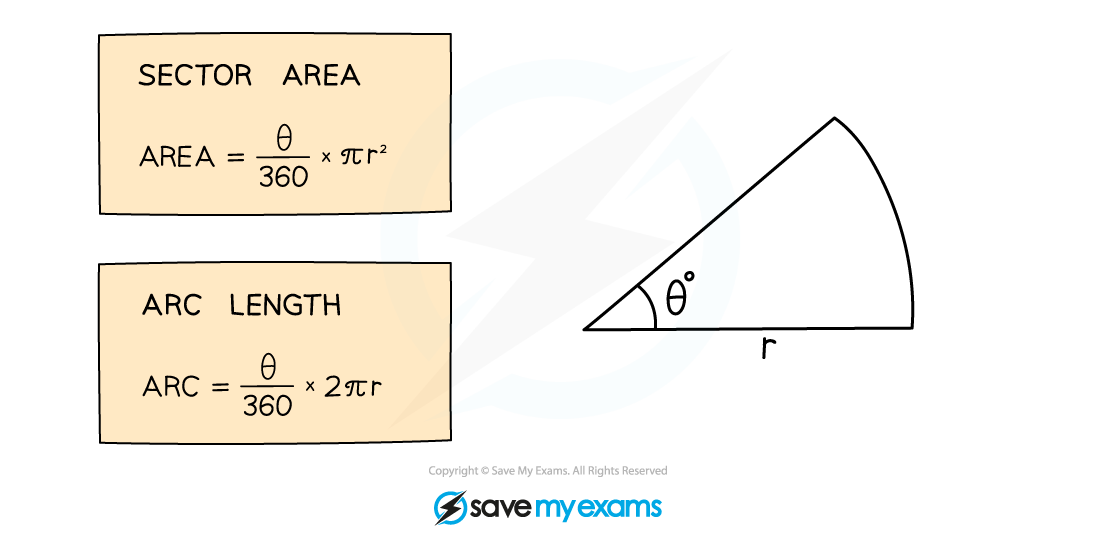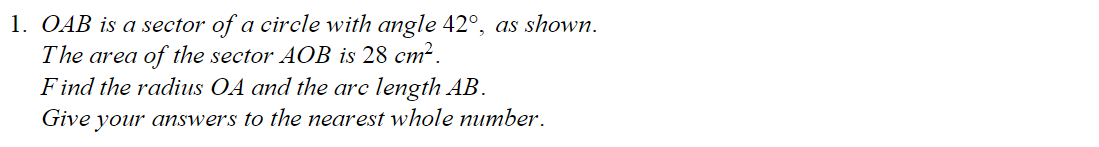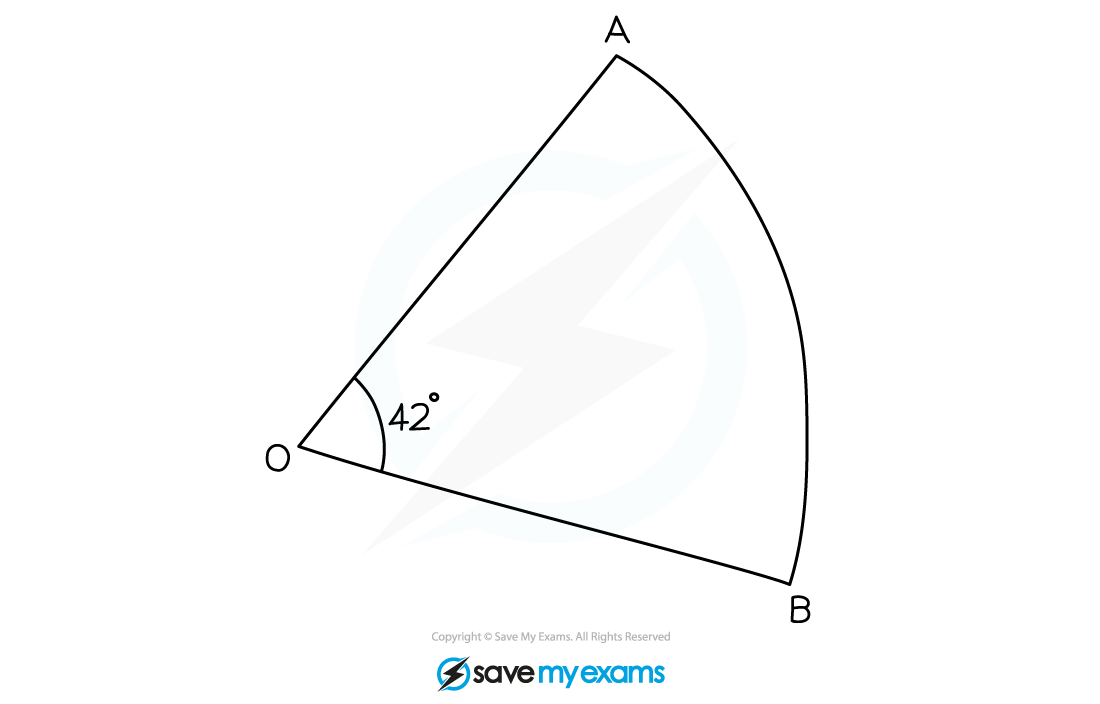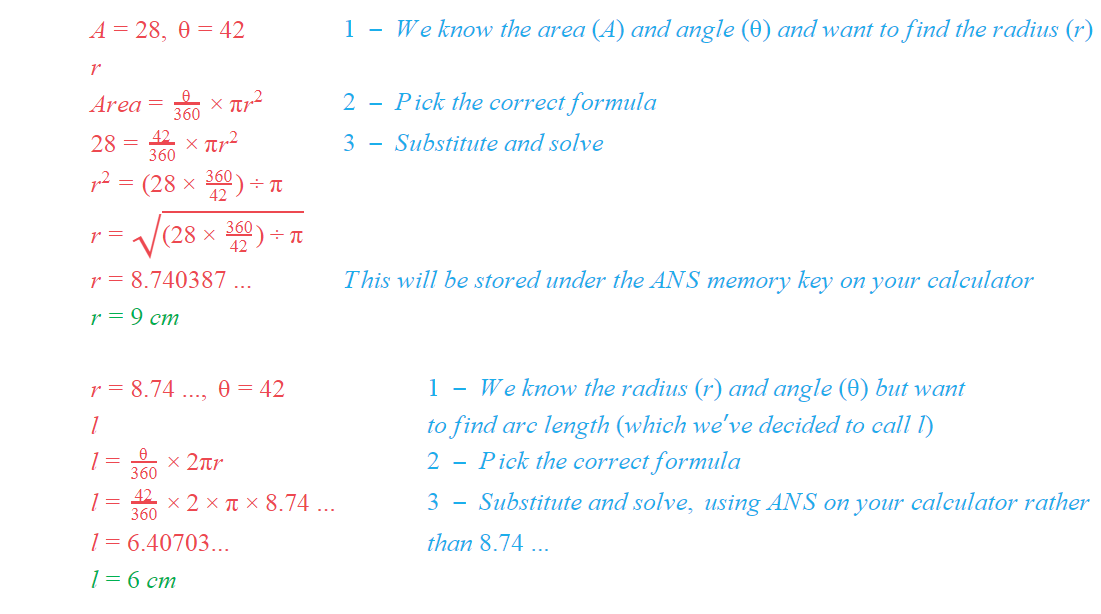# Edexcel IGCSE Maths 复习笔记 4.13.1 Circles - Sector Areas & Arc Lengths

Edexcel IGCSE Maths 复习笔记 4.13.1 Circles - Sector Areas & Arc Lengths

#### What is a sector? What is an arc?

• A maths book will (correctly) tell you that an arc is part of the circumference of a circle and a sector is part of a circle enclosed by two radii (radiuses) and an arc
• It’s much easier to think of a sector as the shape of a slice of a circular pizza (or cake, or pie, or …) and an arc as the curvy bit at the end of it (where the crust is)

• If the angle of the slice is θ (that’s the Greek letter “theta”) then the formulas for the area of a sector and the length of an arc are just fractions of the area and circumference of a circle:• If you are not too good at remembering formulae there is a logic to these two
• You’ll need to remember the circumference and area formulas
• After that we are just finding a fraction of the whole circle – “θ out of 360”
• Other than that, working with sector and arc formulas is just like working with any other formula:

1. WRITE DOWN – what you know (what you want to know)
2. Pick correct FORMULA
3. SUBSTITUTE and SOLVE

#### Exam Tip

If you’re under pressure and can’t remember which formula is which, remember that area is always measured in square units (cm2, m2 etc.) so the formula with r2 in it is the one for area.

#### Worked Example#### Worked Example